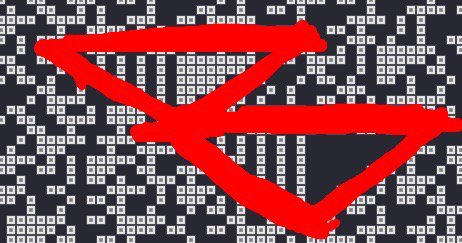# Math puzzle: fibonacci sequence in bits

If you write the Fibonacci sequence using binary representation for each number, a picture like this emerges:Every column of small rectangles is a Fib(N) value, written vertically in binary, with lowest bit at the bottom. Looking at the picture, it is quite clear that the dependency between N and the number of bits to represent Fib(N) is linear. I’m curious though, how to mathematically prove that… (I’m sure @LoopSpace can do that in like 5 seconds, but still…)

Code is here, if anyone needs it:

``````
--# Main
-- Fibobits

displayMode(OVERLAY)
displayMode(FULLSCREEN)

-- Use this function to perform your initial setup
function setup()
A,B = 0,WIDTH/5
bitSize = 5
i = 0

nums = {{ 1 }, { 1 }}
for i=3,B do
nums[i] = add(nums[i-2], nums[i-1])
end
print("prepped: " .. B)
end

local c = {}
local n = math.max(#a,#b)
local carry = 0
for i=1,n do
c[i] = (a[i] or 0) + (b[i] or 0) + carry
if c[i] > 1 then
carry = 1
c[i] = c[i] - 2
else
carry = 0
end
end
if carry > 0 then
c[n+1] = 1
end
return c
end

-- This function gets called once every frame
function draw()
-- This sets a dark background color
background(40, 40, 50)

-- This sets the line thickness
strokeWidth(2)

-- Do your drawing here
if fibImg then
translate(WIDTH/2, HEIGHT/2)
sprite(fibImg)
else
pushStyle()
text("Preparing image ...", WIDTH/2, HEIGHT/2)
stroke(85, 83, 140, 255)
noFill()
rect(WIDTH/2 - 150, HEIGHT/2 - 50, 300, 20)
fill(95, 92, 111, 255)
noStroke()
rect(WIDTH/2 - 150, HEIGHT/2 - 50, 300*i/(B-A), 20)
popStyle()

co = co or coroutine.create(function()
local img = image(WIDTH, HEIGHT)
local count = 0
local s = bitSize
setContext(img)
i = 1
while i<=B-A do
local b = nums[i+A]
for j=1,#b do
count = count + 1
if b[j] == 1 then
rect(i*s,j*s,s,s)
end
if count % 1000 == 0 then
setContext()
coroutine.yield()
setContext(img)
end
end
i = i + 1
end
fibImg = img
setContext()
end)
coroutine.resume(co)
end
end
``````

@Juce Very interesting.

@Juce I looked up Fibonacci linear and it took me to this site. This might explain it being linear.

``````http://mathworld.wolfram.com/FibonacciNumber.html
``````

@dave1707, thanks for the link - very good article. They even have the same picture! They don’t mention the linear nature of the graph, perhaps it is obvious from other formulas and facts… I shall read it carefully

( My thinking so far was like this: to represent a number X, you need Log2(X) bits. In our case X = Fib(N), so for the dependency to be linear, Fib(N) needs to be something 2^(k * N), where k is some constant. Then we have Log2(2^(k * N)) = k * N. But Fib(N) is not 2^(k * N)… So i’m confused… )

@Juce I haven’t read the article yet, but it looks interesting. What kind of picture do you get if you used prime numbers.

@Juce I tried running your code with a bitSize=.5 and B=WIDTH*2 . Still nice and linear.

Fib(N) is 2^(k N), or as near as makes no difference.

More accurately, the numbers in any Fibonacci-like sequence are of the form a phi^n + b phi^{-n} where a and b are constants and phi is the golden ratio. (By “Fibonacci-like” I mean a sequence generated using the same rule as “the” Fibonacci sequence but with possibly different starting points.)

The first crucial point is that phi^{-n} quickly becomes very small. So Fib(n) is approximately a phi^n. In fact, a quick way to calculate Fib(n) is to take the nearest integer to a phi^n.

Thus log_2 Fib(n) is approximately log_2 (a phi^n) which is log_2 a + n log_2 phi.

This is linear. It’s slightly offset by log_2 a, but for the actual Fibonacci sequence then a is 1/sqrt(5) so log_2 of that is around -1. Also, log_2 phi is a bit over 1/2. So the equation is roughly -1 + n/2. The -1 offset is so small you probably don’t notice it.

nice ! And how do explain those recurring structures?@Jmv38, those are cool, indeed! Many of those triangles there…

@LoopSpace, thanks for the explanation. Makes sense. I’m curious how the formula for Fib(n) with powers of phi is derived - i’ll read up on that.

Fibonacci-like sequences are generated by F_{n+2} = F_{n+1} + F_n. We assume that there is a formula of the for F_n = r^n for some r.

Substituting in, we get r^{n+2} = r^{n+1} + r^n. There’s a common factor of r^n in that, and dividing through we get r^2 = r + 1. There are two numbers that satisfy that: phi and 1/phi (where phi is the golden ratio).

So every Fibonacci sequence is of the form: a phi^n + b phi^{-n}. Then you just need to choose a and b so that the first two terms are the first two terms of your sequence.

The standard Fibonacci sequence starts 1,1, and traditionally we start counting at 0, so we choose a and b so that:

a + b = 1

a phi^1 + b phi^{-1} = 1

This leads to the coefficients as stated, since phi = (1 + sqrt(5))/2

this demonstration does not prove that

So every Fibonacci sequence is of the form: a phi^n + b phi^{-n}.

it proves only that there are sequences of this form. Some more is needed to prove every sequence is of this form.

Pick a Fibonacci sequence. Every Fibonacci sequence is completely determined by its first two values. There’s an a and b so that a phi^n + b phi^{-n} starts with those same two values (that’s not hard to show). Since a phi^n + b phi^{-n} is a Fibonacci sequence with the same first two values as our original sequence, it must therefore be the one we’re thinking of.

that’s the proof i was missing. Thanks.